Question

Fluid Mechanics

Problem 5: Pipe Flow

This semester we worked through several versions of the pipe flow problem sketched above, in which a 3.0 ft diameter pipe is used to convey water from reservoir A (surface elevation 80 ft) to reservoir D (surface elevation 90 ft). In week 14, we considered a specific example with a cast iron pipe (A = 0.00085 ft), flow rate of Q = 40 ft/s, and average velocity of V = 5.66 ft/s. For these conditions, the friction factor was 2= 0.015 and the pump added 85 ft of head. Assume the water temperature is always 60°F.

Part A Assuming the pump is 75% efficient and electricity costs 10¢/kW hr, how much will it cost to run this pumpfor a year? Hint, 1 kW hr = (1000 watts)(1 hr) =(1000 J/s)(3600 s) = 3.6x10° J.

Part BNow let us assume we need to double the discharge to Q=80 ft’/s, but unlike the example in week 13, we are not going to make the false assumption that the friction factor 1 is constant. You may, however, assume that the roughness height A is constant. We will consider three options:

Option #1: Build a parallel system with the same pipe and pump, with a flow rate of Q= 40 ft’/sin each system. What is the annual electricity cost?

Option #2: Use the original pipe but double the velocity. What is the annual electricity cost?

Part C Briefly comment on the pros and cons of options 1-3 (<30 words).

Option #3: Build a new pipe with diameter of 50.9 in. The cross-sectional area of this pipe is twice that of a 36 in pipe, so the velocity will be V= 5.66 ft/s for a discharge of Q= 80 ft’/s.What is the annual electricity cost?Verified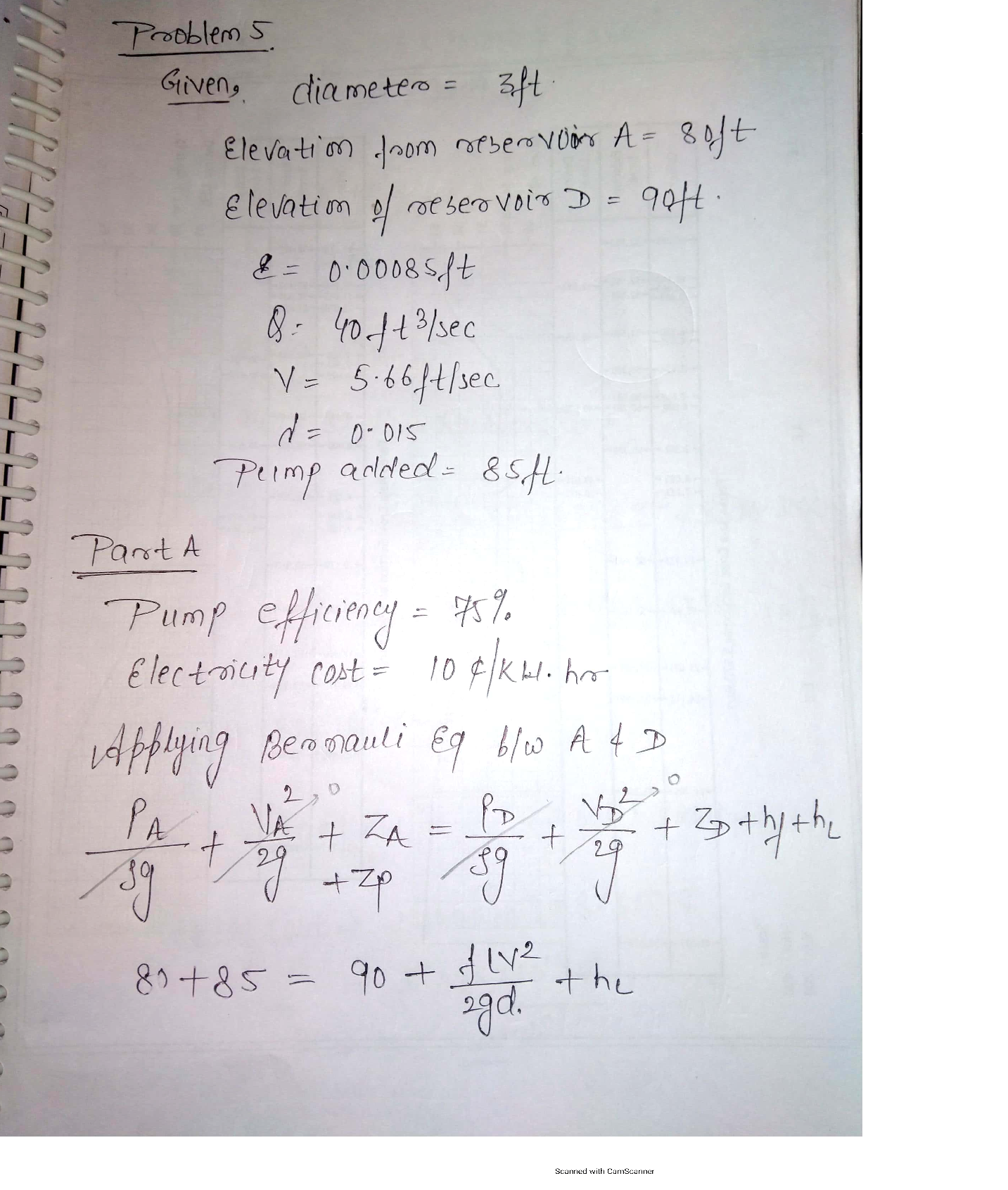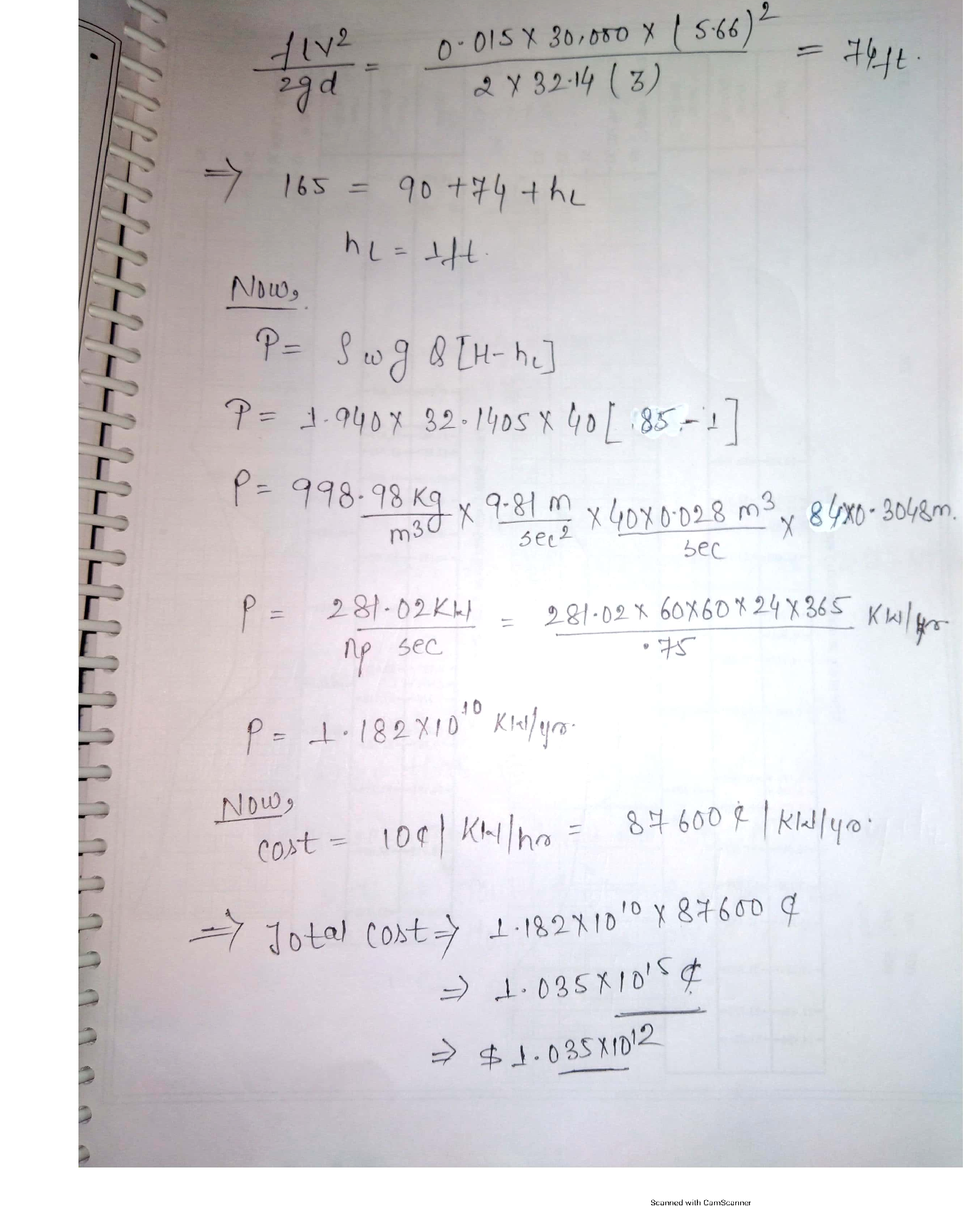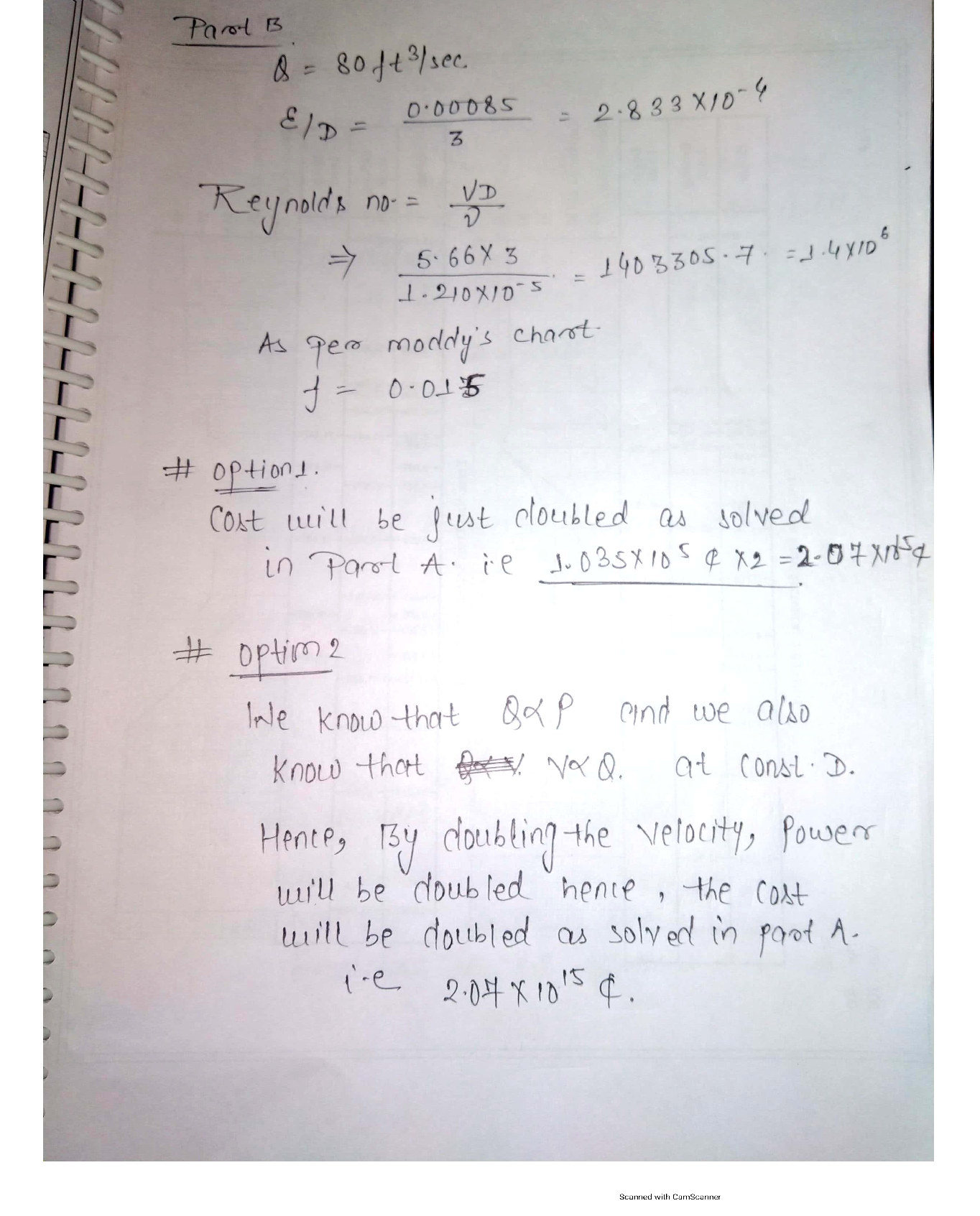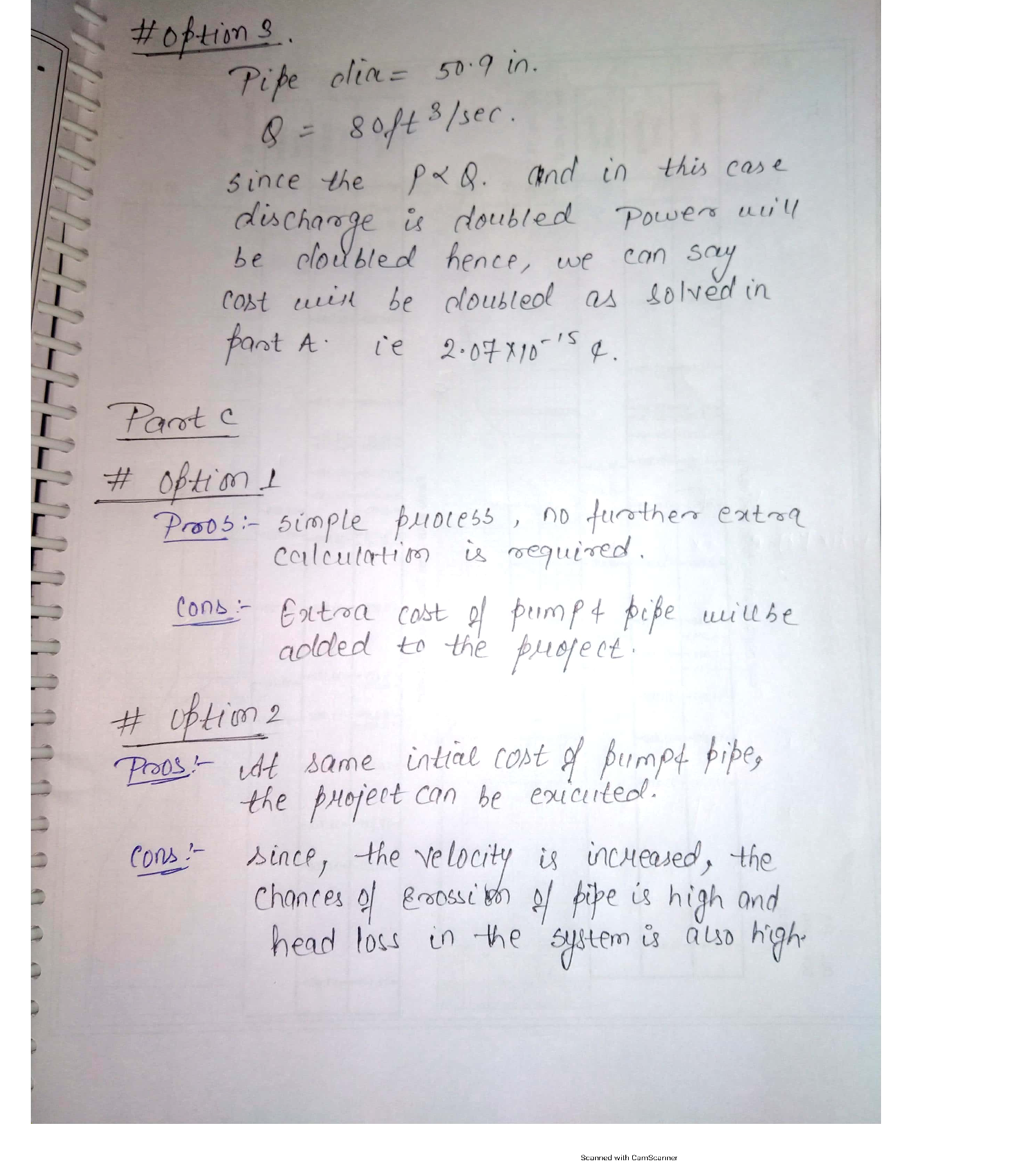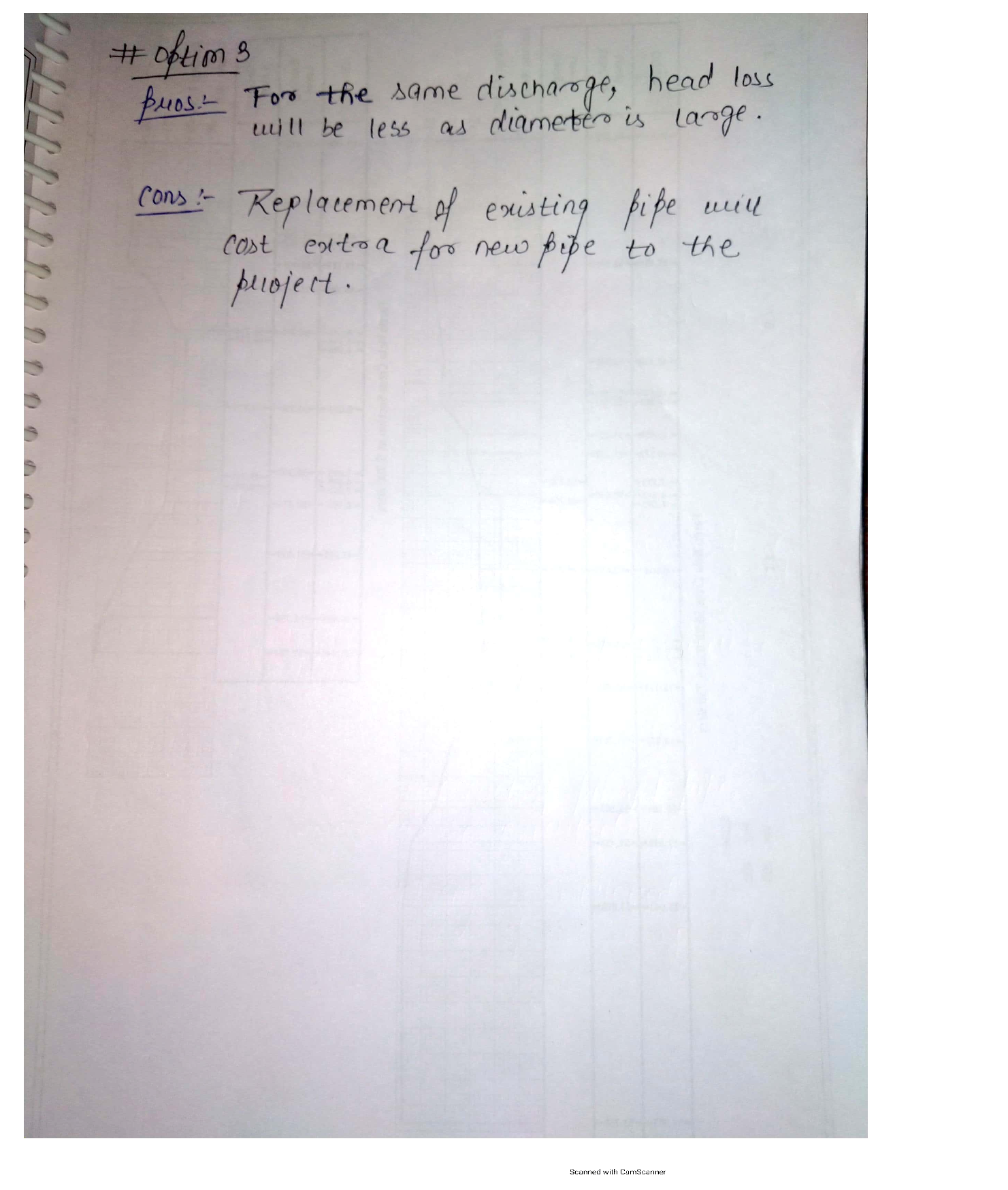### Question 45484Fluid Mechanics

Water in the Belle Fourche Reservoir in Western South Dakotais transported to the water storage reservoir for the town of-Buffalo through a series of three pipes, all with friction factors of 0.02. The first pipe is 500 meters long with a diameter of 0.4meters; the second pipe is 600 meters long with a diameter of0.3 meters; and the third pipe is 500 meters long with a diameter of 0.2 meters. The water surface elevation of the Belle FourcheReservoir is 30 meters higher than the town's storage reservoir.Since the current piping is almost 60 years old, the town of Buffalo has decided to replace the piping system with a single pipe of constant diameter. You have been chosen to calculate the equivalent diameter for the single pipe design and determine the flow rate that will occur. Go to work.

### Question 44899Fluid Mechanics

Transmission lines

### Question 44896Fluid Mechanics

A boat has a volume of 40.0 m³ and a mass of 2.00 tons. What load will push 75.0% of its volume into water? Each Metric ton is 1000 kg.

### Question 44895Fluid Mechanics

A tank has a height of 800 mm and a length of 500 mm. The tank contains a liquid of RD 0.9.Determine:
a. The magnitude of the force on the side of the tank per meter width and its position relative to the base of the tank
b. The force on the base per meter width of tank.

### Question 44894Fluid Mechanics

A closed tank contains three different fluids with a vapor space above the oil, as shown in the figure below. Pressure gauge 1 reads the gauge pressure of the vapor space (P1) and pressure gauge 2 reads the pressure at the bottom of the tank (P2). When P1 = 25 kPa, determine:
a. Height of glycerine (h) in the tank if P2 = 95.66 kPa
. P2 reading if glycerine is replaced with water

### Question 44805Fluid Mechanics

Water flows into cylindrical tank through inlet A and D at rate of 20 ft/s and 5 ft/s respectively. If waterleaves through outlet B and C at rate of 3 ft/s and 10 ft/s respectively.
(A) Derive the continuity equation
\text { Know that } D_{A}=2.5 \text { in, } D_{D}=2 \text { in } D_{B}=3 \text { in and } D_{C}=3.5 \text { in. }
(B) Calculate the change of high h?

### Question 44804Fluid Mechanics

An object which has cubic shape is been floating on the water surface see the diagram below. If the object density is less than water, density find the ratio X/L?

### Question 44803Fluid Mechanics

A force of 15.83 kN is exerted on a piston B (diameter = 250 mm) which fits into a cylinder see the diagram below. How much force is need on piston C to start displacing piston B and Mercury on piston B?

### Question 44802Fluid Mechanics

Given the diagram below if the pressure gauge reads 6.1 psig find Y and X in ft. Given that
\mathrm{SG}_{\mathrm{Aloohal}}=0.9, \mathrm{SG}_{\mathrm{Hg}}=13.6, \text { Alcohol vapor } P=1.7 \mathrm{psig}, Z=4 \mathrm{ft} \text { and } \mathrm{Y}_{\mathrm{H} 2 \mathrm{o}}=62.4 \mathrm{\textrm {lb }} / \mathrm{ft}^{3}

### Question 44801Fluid Mechanics

Given the diagram below, calculate the pressure at A, B, C.
\text { Given that } \mathrm{Y} \text { н2о }=9.79 \mathrm{kN} / \mathrm{m}

### Submit query